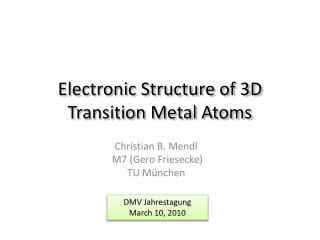Download PresentationElectronic Structure of 3D Transition Metal Atoms# Electronic Structure of 3D Transition Metal Atoms - PowerPoint PPT Presentation

Download Presentation##### Electronic Structure of 3D Transition Metal Atoms

Download Policy: Content on the Website is provided to you AS IS for your information and personal use and may not be sold / licensed / shared on other websites without getting consent from its author. While downloading, if for some reason you are not able to download a presentation, the publisher may have deleted the file from their server.

- - - - - - - - - - - - - - - - - - - - - - - - - - - E N D - - - - - - - - - - - - - - - - - - - - - - - - - - -
##### Presentation Transcript

1. Electronic Structure of 3D Transition Metal Atoms Christian B. Mendl M7 (GeroFriesecke) TU München DMV Jahrestagung March 10, 2010

2. Outline • basic setting: Schrödinger equation for an N-electron atom, configuration-interaction (CI) model • this talk: algorithmic framework, up to N = 30 electrons • naïve approch: • main ingredient: efficient simultaneous angular momentum and spin diagonalization (prior to setting up the CI Hamiltonian) GeroFriesecke and Christian B. Mendl. An algorithmic framework for electronic structure calculations of 3D transition metal atoms. to be published (2010)

3. QM Framework time-independent, nonrelativistic Schrödinger equation with N number of electrons Z > 0 nuclear charge single particle Hamiltonian: kinetic energy and external nuclear potential inter-electron Coulomb repulsion

4. Symmetries • invariance under simultaneous rotation of electron positions/spins, sign reversal of positions • → angular momentum, spin and parity operators • N-particle space • pairwise commuting: • → quantum numbers (corresponding to eigenvalues)

5. Configurations • start from irreducible representation spaces for the joint spin and angular momentum algebra in the single-particle L2 space (“atomic subshells”) • abstract definition of “configuration” • invariant under the angular momentum, spin and parity operators L, S, R • example: GeroFriesecke and Benjamin D. Goddard. Asymptotics-based CI models for atoms. SIAM Journal on Multiscale Modeling and Simulation(2009)

6. Asymptotics-Based CI Models • finite-dimensional projection of the Schrödinger equation • retains the spin and angular momentum symmetries of the atomic Schrödinger equation • asymptotics-based → Slater-type orbitals (STOs), correct asymptotic behavior for N fixed and Z→∞ under suitable choice of configurations

7. LS Diagonalization • basic idea: calculate simultaneous angular momentum, spin and parity eigenspaces first before touching the Hamiltonian → huge cost reduction • need tensor product structure to make use of Clebsch-Gordan coefficients, but have to take antisymmetrization constraint into account • → solution via configurations • isometry • observation: isometry respects LS operators, i.e., GeroFriesecke and Christian B. Mendl. An algorithmic framework for electronic structure calculations of 3D transition metal atoms. to be published (2010)

8. LS Diagonalization (cont.) • first step: simultaneous diagonalization of • configurations → • each VI is an invariant subspace of the LS-operators → diagonalization can be performed on each VI independently via Clebsch-Gordan coefficients, • → obtain decomposition into irreducible LS eigenspaces Angelika Bunse-Gerstnert, Ralph Byers, and Volker Mehrmann. Numerical methods for simultaneous diagonalization. SIAM Journal on Matrix Analysis and Applications (1993) such that →

9. Cost Analysis: Configurations • algorithm of order , e.g. simultaneous matrix diagonalization • Stirling’s formula → approximated gain factor (quotient) • independent of particle number N! • example: Chromium with three active subshells (3p,3d,4s) and N = 12 active orbitals, algorithmic order p = 3 → vs. approximated factor: “exact” factor:

10. Cost Analysis: Symmetries • diagonalize H within each LS eigenspace separately • representation theory → need only consider states with quantum numbers (can traverse the Lz and Sz eigenstates by ladder operators L± and S±) • example: Chromium with configurations • full CI dimension: • 7S symmetry level 14 states only

11. Results goal: derive the anomalous filling order of Chromium from first principles quantum mechanics • green: experimental ground state symmetry • blue: the lower of each pair of energies • exact agreement with experimental data! • additional ideas used: • RDMs • sparse matrix structure • closed-form orthnormalization, Hankel matrices http://sourceforge.net/projects/fermifab